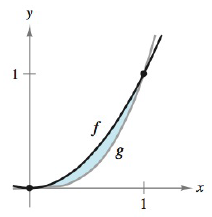Chapter 5.5, Problem 4E### Calculus: An Applied Approach (Min...

10th Edition
Ron Larson
ISBN: 9781305860919

#### Solutions

Chapter
Section### Calculus: An Applied Approach (Min...

10th Edition
Ron Larson
ISBN: 9781305860919
Textbook Problem
1 views

# Finding the Area Bounded by Two Graphs In Exercises 1-8, find the area of the region. See Examples 1, 2, 3, and 4. f ( x ) = x 2 g ( x ) = x 3To determine

To calculate: The area bounded by the function f(x)=x2 and g(x)=x3.

Explanation

Given Information:

The provided function is f(x)=x2 and g(x)=x3 and the provided graph is shown below,

Formula used:

The function f(x) and g(x) are the continuous function on interval [a,b] and g(x)f(x) for all x in [a,b], then, the area bounded by the graphs of f(x) and g(x),x=a and x=b is,

A=ab[f(x)g(x)]dx

Calculation:

Consider the provided function is f(x)=x2 and g(x)=x3.

In the provided function f(x)=x2 and g(x)=x3 where, g(x)f(x)

### Still sussing out bartleby?

Check out a sample textbook solution.

See a sample solution

#### The Solution to Your Study Problems

Bartleby provides explanations to thousands of textbook problems written by our experts, many with advanced degrees!

Get Started

#### In Problems 7-34, perform the indicated operations and simplify. 19.

Mathematical Applications for the Management, Life, and Social Sciences

#### The polar equation of the conic with eccentricity 3 and directrix x = −7 is:

Study Guide for Stewart's Single Variable Calculus: Early Transcendentals, 8th

#### For

Study Guide for Stewart's Multivariable Calculus, 8th

#### Define fraud and explain the safeguards that exist to prevent it.

Research Methods for the Behavioral Sciences (MindTap Course List)